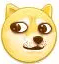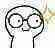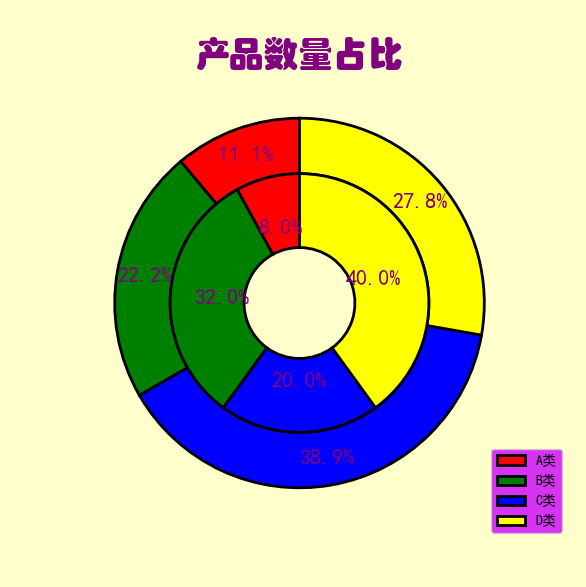# python-matplotlib库绘制饼形图专题(从一般饼状图到内嵌环形图)

python-matplotlib库绘制饼形图专题(从一般饼状图到内嵌环形图)

### 文章目录

• 1.plt.pie()
• 2. 饼图基本
• 3. 饼状图进阶
• 4. 环形图
• 5. 内嵌环形图

•       ʚʕ̯•͡˔•̯᷅ʔɞʚʕ̯•͡˔•̯᷅ʔɞʚʕ̯•͡˔•̯᷅ʔɞʚʕ̯•͡˔•̯᷅ʔɞʚʕ̯•͡˔•̯᷅ʔɞʚʕ̯•͡˔•̯᷅ʔɞʚʕ̯•͡˔•̯᷅ʔɞʚʕ̯•͡˔•̯᷅ʔɞʚʕ̯•͡˔•̯᷅ʔɞʚʕ̯•͡˔•̯᷅ʔɞʚʕ̯•͡˔•̯᷅ʔɞʚʕ̯•͡˔•̯᷅ʔɞʚʕ̯•͡˔•̯᷅ʔɞʚʕ̯•͡˔•̯᷅ʔɞʚʕ̯•͡˔•̯᷅ʔɞʚʕ̯•͡˔•̯᷅ʔɞʚʕ̯•͡˔•̯᷅ʔɞʚʕ̯•͡˔•̯᷅ʔɞ

大家好，我是侯小啾！今天分享的内容是，基于python-matplotlib库的饼形图绘制，具体内容涉及一般的饼图、分裂饼图、以及环形图。

# 1.plt.pie()

饼图 常常用来显示 整体中各部分所占的比例，在python-matplotlib库中通过plt.pie()方法来实现。
其主要参数如下：

x ——–每一块饼图的比例组成的序列。可以是一个列表。如果其中元素的值加起来大于一，则会通过
归一化处理后的结果。

explode ——– 指每一块饼距离圆心的距离。默认为0。如果大于0，则饼即会分裂出来，即“分裂饼形图”效果。

labels ——– 标签，即每一块饼图外侧显示的说明文字

autopct ——– 设置饼图百分比的格式。即字符串的格式，可以使用格式化字符串表达式或者format()函数的表达式。 不设定这个参数则不会显示百分比的字符串。

pctdistance ——–指百分比的位置刻度，默认为0.6。

labeldistance ——–标记的绘制的位置，占半径长度的比例。默认为1.1，如果大于1则显示在饼的内侧。

startangle ——–起始绘制角度，默认从x轴正方向逆时针画起。例如，设置为90则从y轴正方向画起。

counterclock ——– 指针方向。布尔类型。默认为True，表示逆时针。如果为False则表示顺时针。

wedgeprops ——– wedge对象的属性（wedge翻译过来即三角木，楔子，即表示饼图中的每一块儿），可选参数，字典类型，默认为None。这个字典将会传递给wedge对象。如wedgeprops={‘linewidth’:2}表示wedge的线条宽度为2。

textprops ——– text标签文本的属性。也是一个字典类型，可选。默认为None。

center ——– 饼图的原点。

frame ——– 是否显示轴框架，布尔类型，默认不显示，False。如果为True则会显示轴框架。可以与grid()配合使用。通常不使用该参数，因为轴框架会干扰图像效果。

rotatelabels ——– 可选，旋转标签到指定角度。（“指定”的含义是，以该位置连接圆心的半径为水平基准。）

# 2. 饼图基本

绘制一张简单的饼图，图像上的百分数显示出一位小数。标签为[‘A类’, ‘B类’, ‘C类’, ‘D类’]。

``````import matplotlib.pyplot as plt
plt.rcParams['font.sans-serif'] = ['SimHei']
plt.rcParams['axes.unicode_minus'] = False
plt.rcParams['axes.facecolor'] ='#cc00ff'
x = [10, 20, 35, 25]
plt.pie(x, autopct='%1.1f%%', labels=['A类', 'B类', 'C类', 'D类'])
plt.title("产品数量占比")
plt.show()
``````

图像效果呈现如下：# 3. 饼状图进阶

设置背景颜色为’#00E5EE’，
百分数显示一位小数，
四块的颜色依次为[‘red’, ‘green’, ‘purple’, ‘yellow’]
B类 在饼图中 向外分裂0.2个单位  explode=[0, 0.2, 0, 0]，
从y轴正方向开始绘图  startangle=90，
标签文本字体的大小为20，颜色为 ‘#8B1A1A’    textprops={‘fontsize’: 20, ‘color’: ‘#8B1A1A’}
显示阴影效果，
标签在半径0.6单位处    pctdistance=0.6，
每个标签都选择一定的角度   rotatelabels=True

代码如下：

``````import matplotlib.pyplot as plt
fig = plt.figure(1, facecolor='#00E5EE', figsize=(10, 8))
plt.rcParams['font.sans-serif'] = ['SimHei']
plt.rcParams['axes.unicode_minus'] = False
plt.rcParams['axes.facecolor'] = '#cc00ff'
x = [10, 20, 35, 25]
plt.pie(x,
labels=['A类', 'B类', 'C类', 'D类'],
autopct='%1.1f%%',
colors=['red', 'green', 'purple', 'yellow'],
explode=[0, 0.2, 0, 0],
startangle=90,
textprops={'fontsize': 20, 'color': '#8B1A1A'},
pctdistance=0.6,
rotatelabels=True
)
# 设置标题文本、y坐标（即竖直方向上的位置），字体大小、颜色
plt.title("产品数量占比", y=1.05, fontsize=25, color='#cc00ff')
plt.show()
``````# 4. 环形图

绘制环形图仍然使用plt.pie()方法，
只需要设置其中的wedgeprops参数的属性。

代码示例如下
在上例代码的基础上，取消分裂，
添加 wedgeprops={‘width’: 0.4, ‘edgecolor’: ‘k’}。
然后把百分数的位置pctdistance设置为0.8。

``````import matplotlib.pyplot as plt
fig = plt.figure(1, facecolor='#00E5EE', figsize=(10, 8))
plt.rcParams['font.sans-serif'] = ['SimHei']
plt.rcParams['axes.unicode_minus'] = False
plt.rcParams['axes.facecolor'] = '#cc00ff'
x = [10, 20, 35, 25]
plt.pie(x,
labels=['A类', 'B类', 'C类', 'D类'],
autopct='%1.1f%%',
colors=['red', 'green', 'purple', 'yellow'],
startangle=90,
textprops={'fontsize': 20, 'color': '#8B1A1A'},
pctdistance=0.8,
rotatelabels=True,
wedgeprops={'width': 0.4, 'edgecolor': 'k'}
)
plt.title("产品数量占比", y=1.05, fontsize=25, color='#cc00ff')
plt.show()
``````

代码执行效果如下：# 5. 内嵌环形图

内嵌环形图，以内嵌一次为例。即两个圆环，需要调用两次plt.pie()方法。

全局字体为SimHei（黑体）。标题部分的局部字体使用“华文琥珀”（将系统的字体文件STHUPO.TTF复制到当前目录下）。

``````import matplotlib.pyplot as plt
from matplotlib import font_manager

fig = plt.figure(1, facecolor='#ffffcc', figsize=(6, 6))
plt.rcParams['font.sans-serif'] = ['SimHei']
plt.rcParams['axes.unicode_minus'] = False
plt.rcParams['axes.facecolor'] = '#cc00ff'
x1 = [10, 20, 35, 25]
x2 = [8, 32, 20, 40]
plt.pie(x1,
autopct='%1.1f%%',
colors=['red', 'green', 'blue', 'yellow'],
startangle=90,
textprops={'fontsize': 16, 'color': 'purple'},
pctdistance=0.85,
rotatelabels=True,
wedgeprops={'linewidth': 2, 'width': 0.3, 'edgecolor': 'k'}
)
plt.pie(x2,
autopct='%1.1f%%',
colors=['red', 'green', 'blue', 'yellow'],
startangle=90,
textprops={'fontsize': 16, 'color': 'purple'},
pctdistance=0.6,
rotatelabels=True,
wedgeprops={'linewidth': 2, 'width': 0.4, 'edgecolor': 'k'}
)
plt.title("产品数量占比", fontsize=25, color='purple', fontproperties=font_manager.FontProperties(fname='STHUPO.TTF'))
plt.legend(['A类', 'B类', 'C类', 'D类'], bbox_to_anchor=(0.9, 0.2))
plt.show()
``````

程序执行效果如下：本次分享就到这里，小啾感谢您的关注与支持！
🌹꧔ꦿ🌹꧔ꦿ🌹꧔ꦿ🌹꧔ꦿ🌹꧔ꦿ🌹꧔ꦿ🌹꧔ꦿ🌹꧔ꦿ🌹꧔ꦿ🌹꧔ꦿ🌹꧔ꦿ🌹꧔ꦿ🌹꧔ꦿ🌹꧔ꦿ🌹꧔ꦿ🌹꧔ꦿ🌹꧔ꦿ🌹꧔ꦿ🌹꧔ꦿ🌹꧔ꦿ🌹꧔ꦿ🌹꧔ꦿ🌹꧔ꦿ🌹꧔ꦿ🌹꧔ꦿ🌹꧔ꦿ🌹꧔ꦿ🌹꧔ꦿ

来源：侯小啾